## 54) Bridging Ten Subtraction

Ten Plus Bonds: Strategy Adding On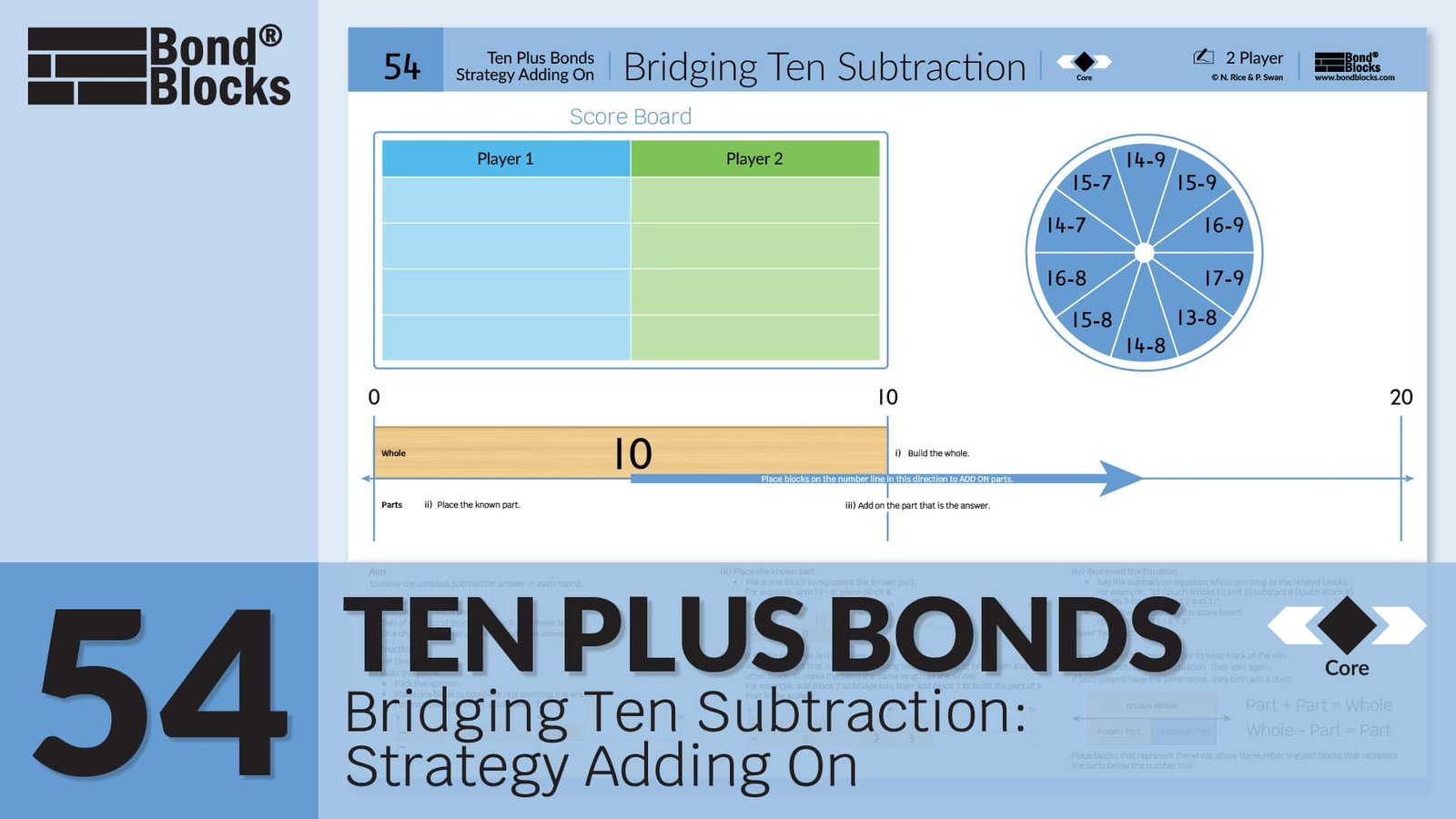### Mathematics

Develop the strategy of bridging ten to subtract, using adding on, for wholes up to 20.

For example, to solve 13 – 8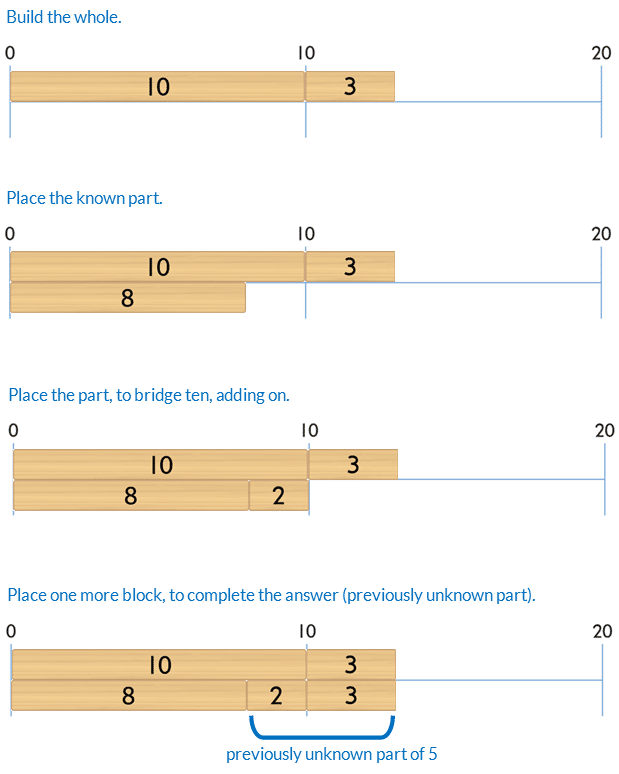Prior knowledge of part-part-whole, bonds of 10 and place value partitioning using ten plus bonds is needed.### Language

• bridge ten
• subtract
• equals
• right

Note, in this activity students are finding a missing part in a subtraction equation.

They are NOT completing “sums”. The “sum” is the whole, found by adding the parts.

The word ‘sum’ is often incorrectly used when the word ‘equation’ is meant. It is impossible to “complete a subtraction sum”.## Differentiation

### A little easier

##### Counting order

Calculate each question in counting order, working clockwise around the spinner, from the 12 o’clock position. Do not flick the spinner. Students complete each type of equation:

• Subtract 9
• Subtract 8
• Subtract 7

Assist students to identify patterns of adding on 1, 2 or 3 depending on the part being subtracted.

##### Use Bond Blocks

If students have difficulty partitioning the part being taken away to bridge ten, use an additional block.

For example, to model 13 – 8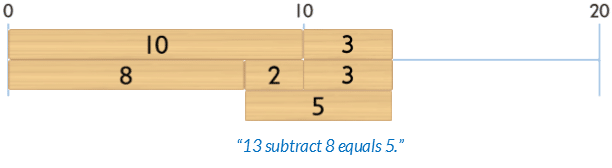Some students find it conceptually easier to place the block representing the part being added on, that is the answer to the subtraction. Place this block on top of this block of the block that was used to bridge ten and the other block needed to make the part that is the answer.

• From a top view they can see the parts of 8 and 5.
• From a front view they can see the partition of 2 and 3.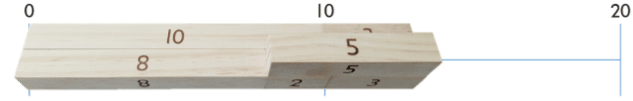##### Directional difficulties

Click to download a Comparison and Number line Language: Desk Visual to support students who have directional and sequencing difficulties.### A little harder

##### Bridging ten subtraction strategy taking away

Play “Ten Plus Bonds: Bridging Ten Subtraction Strategy Adding On a little harder”. In this game students have to solve subtraction by bridging ten, to twenty, for wholes up to 30.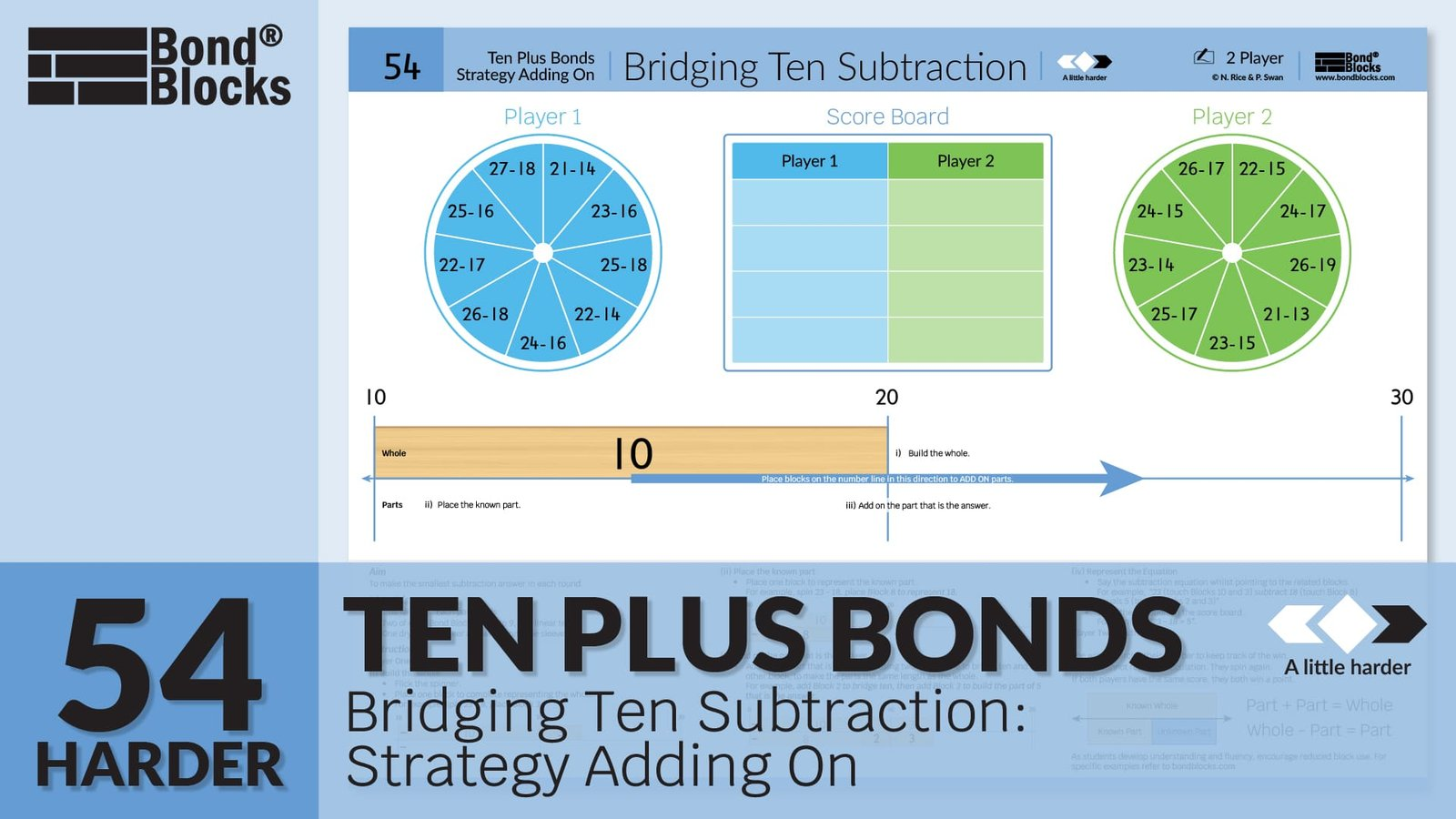##### Reduce scaffolds

The blocks are a scaffold to support calculation. If students can calculate without some or all of the blocks encourage this. Below is a progression of reducing scaffolding, from most to least support, using bridging ten for 23-18.##### Choosing equations

Instruct students to write one subtraction equation, with a whole less than 20, that can be solved efficiently using adding on (adding on to the known part). Students model this with Bond Blocks to explain their reasoning.

Repeat for subtraction solved using taking away.

Discuss which types of questions are more suited to each type of subtraction and why.

### Progression

In the next activity students develop another strategy for adding numbers to 20, partitioning using five plus bonds. Go to

##### Activity 55

Ten Plus Bonds: Partitioning Addition, Strategy Five Plus Bonds